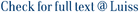Indexing highly repetitive texts - such as genomic databases, software repositories and versioned text collections - has become an important problem since the turn of the millennium. A relevant compressibility measure for repetitive texts is r, the number of runs in their Burrows-Wheeler Transform (BWT). One of the earliest indexes for repetitive collections, the Run-Length FM-index, used O(r) space and was able to efficiently count the number of occurrences of a pattern of length m in the text (in loglogarithmic time per pattern symbol, with current techniques). However, it was unable to locate the positions of those occurrences efficiently within a space bounded in terms of r. Since then, a number of other indexes with space bounded by other measures of repetitiveness - the number of phrases in the Lempel-Ziv parse, the size of the smallest grammar generating the text, the size of the smallest automaton recognizing the text factors - have been proposed for efficiently locating, but not directly counting, the occurrences of a pattern. In this paper we close this long-standing problem, showing how to extend the Run-Length FM-index so that it can locate the occ occurrences efficiently within O (r) space (in loglogarithmic time each), and reaching optimal time O (m + occ) within O (r log(n/r)) space, on a RAM machine with words of w = Omega(log n) bits. Raising the space to O (rw log(sigma) (n/r)), we support locate in O (m log(sigma) =w + occ) time, which is optimal in the packed setting and had not been obtained before in compressed space. We also describe a structure using O (r log(n/r)) space that replaces the text and efficiently extracts any text substring, with an O (log(n/r)) additive time penalty over the optimum. Preliminary experiments show that our new structure outperforms the alternatives by orders of magnitude in the space/time tradeoff map.

Optimal-Time Text Indexing in BWT-runs Bounded Space / Gagie, T; Navarro, G; Prezza, Nicola. - SODA '18: Proceedings of the Twenty-Ninth Annual ACM-SIAM Symposium on Discrete Algorithms, (2018), pp. 1459-1477. (Twenty-Ninth Annual ACM-SIAM Symposium on Discrete Algorithms, New Orleans, Louisiana, January, 2018).

### Optimal-Time Text Indexing in BWT-runs Bounded Space

#### Abstract

Indexing highly repetitive texts - such as genomic databases, software repositories and versioned text collections - has become an important problem since the turn of the millennium. A relevant compressibility measure for repetitive texts is r, the number of runs in their Burrows-Wheeler Transform (BWT). One of the earliest indexes for repetitive collections, the Run-Length FM-index, used O(r) space and was able to efficiently count the number of occurrences of a pattern of length m in the text (in loglogarithmic time per pattern symbol, with current techniques). However, it was unable to locate the positions of those occurrences efficiently within a space bounded in terms of r. Since then, a number of other indexes with space bounded by other measures of repetitiveness - the number of phrases in the Lempel-Ziv parse, the size of the smallest grammar generating the text, the size of the smallest automaton recognizing the text factors - have been proposed for efficiently locating, but not directly counting, the occurrences of a pattern. In this paper we close this long-standing problem, showing how to extend the Run-Length FM-index so that it can locate the occ occurrences efficiently within O (r) space (in loglogarithmic time each), and reaching optimal time O (m + occ) within O (r log(n/r)) space, on a RAM machine with words of w = Omega(log n) bits. Raising the space to O (rw log(sigma) (n/r)), we support locate in O (m log(sigma) =w + occ) time, which is optimal in the packed setting and had not been obtained before in compressed space. We also describe a structure using O (r log(n/r)) space that replaces the text and efficiently extracts any text substring, with an O (log(n/r)) additive time penalty over the optimum. Preliminary experiments show that our new structure outperforms the alternatives by orders of magnitude in the space/time tradeoff map.
##### Scheda breve Scheda completa Scheda completa (DC)2018
File in questo prodotto:
File
optimal-time text indexing.pdf

Solo gestori archivio

Tipologia: Versione dell'editore
Licenza: DRM (Digital rights management) non definiti
Dimensione 445.99 kB
Utilizza questo identificativo per citare o creare un link a questo documento: `https://hdl.handle.net/11385/194121`
•59
•33